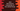# Java program to find union and intersection of two sorted Arrays## Java program to find Union and Intersection of two sorted arrays :

In this tutorial, we will find the union and intersection of two sorted array elements. Let’s find out the union first :

### Union of two sorted arrays using Java :

1. Scan both arrays simultaneously
2. Compare element of both of the array and print the smaller value.
3. Increment the counter of the array with the smaller value.
4. After smaller array is completed. Print out the remaining elements of the larger array.

### Source code :

``````public class Main {

public static void main(String[] args) {
int firstArr[] = {1, 2, 3, 4, 5, 6};
int secondArr[] = {4, 9, 13, 15, 16, 17};

findUnion(firstArr, secondArr);
}

private static void findUnion(int[] firstArr, int[] secondArr) {
int i = 0;
int j = 0;

while (i < firstArr.length && j < secondArr.length) {
if (firstArr[i] < secondArr[j]) {
System.out.print(firstArr[i] + " ");
i++;
} else if (secondArr[j] < firstArr[i]) {
System.out.print(secondArr[j] + " ");
j++;
} else {
System.out.print(firstArr[i] + " ");
i++;
j++;
}
}

while (i < firstArr.length) {
System.out.print(firstArr[i] + " ");
i++;
}

while (j < secondArr.length) {
System.out.print(secondArr[j] + " ");
j++;
}
}

}``````

### Intersection of two sorted arrays using Java :

1. Scan both arrays simultaneously
2. Compare element of both of the array.
3. If both of the values same, print it.
4. Else, increment the counter of the array with smaller element.

### Source Code :

``````public class Main {

public static void main(String[] args) {
int firstArr[] = {1, 2, 3, 4, 5, 6};
int secondArr[] = {4, 9, 13, 15, 16, 17};

findIntersection(firstArr, secondArr);
}

private static void findIntersection(int[] firstArr, int[] secondArr) {
int i = 0;
int j = 0;

while (i < firstArr.length && j < secondArr.length) {
if (firstArr[i] < secondArr[j]) { i++; } else if (firstArr[i] > secondArr[j]) {
j++;
} else {
System.out.print(firstArr[i] + " ");
i++;
j++;
}
}
}

}``````